# AKT-14/Homework Assignment 1

This assignment is due in class on Monday January 20. Here and everywhere, neatness counts!! You may be brilliant and you may mean just the right things, but if the your readers will be having hard time deciphering your work they will give up and assume it is wrong.

This assignment was written on the HW session of Friday January 10. See BBS/AKT14-140110-191441.jpg, BBS/AKT14-140110-192041.jpg, and BBS/AKT14-140110-192042.jpg.

Question 1.

A. Prove that the set of all 3-colourings of a knot diagram is a vector space over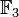${\mathbb F}_3$. Hence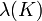$\lambda(K)$ is always a power of 3.

B. Prove that$\lambda(K)$ is computable in polynomial time in the number of crossings of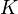$K$.

Question 2. Using combinatorics alone prove that given a 2-component link diagram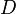$D$, with components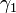$\gamma_1$ and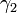$\gamma_2$,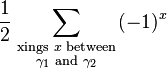$\frac12\sum_{\scriptstyle\text{xings }x\text{ between}\atop\scriptstyle\gamma_1\text{ and }\gamma_2}(-1)^x$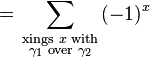$= \sum_{\scriptstyle\text{xings }x\text{ with}\atop\scriptstyle\gamma_1\text{ over }\gamma_2}(-1)^x$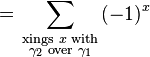$= \sum_{\scriptstyle\text{xings }x\text{ with}\atop\scriptstyle\gamma_2\text{ over }\gamma_1}(-1)^x$

Question 3. Use the techniques from Friday January 10, along with that Friday's handout (the quantum pendulum), to write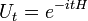$U_t=e^{-itH}$, with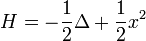$H=-\frac12\Delta+\frac12x^2$, in explicit terms, for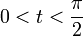$0. Note that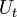$U_t$ interpolates between the identity and the Fourier transform.